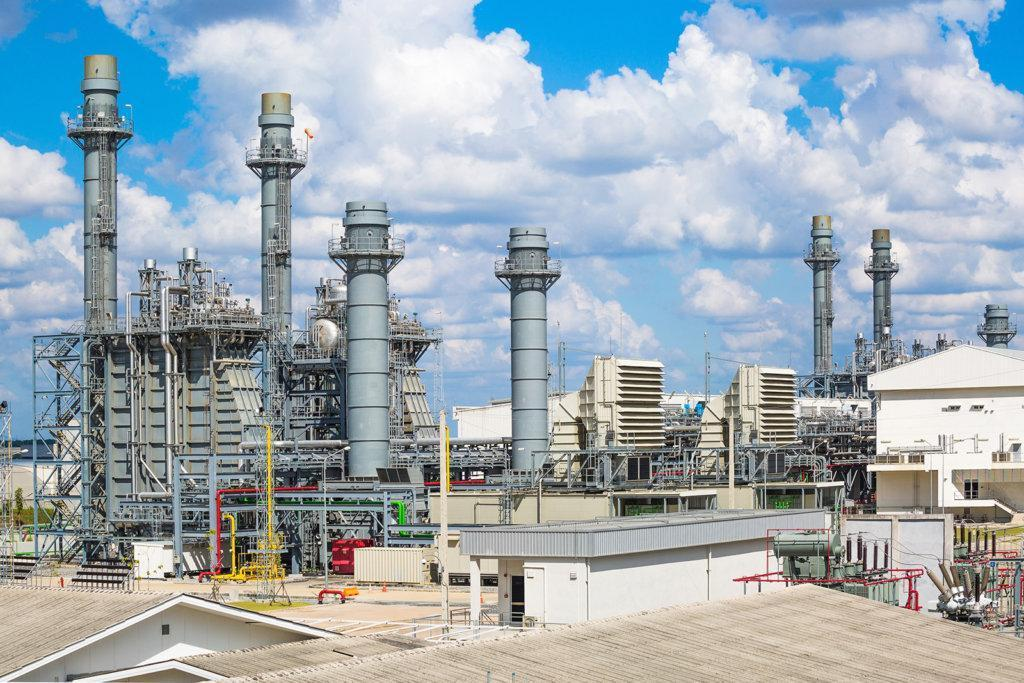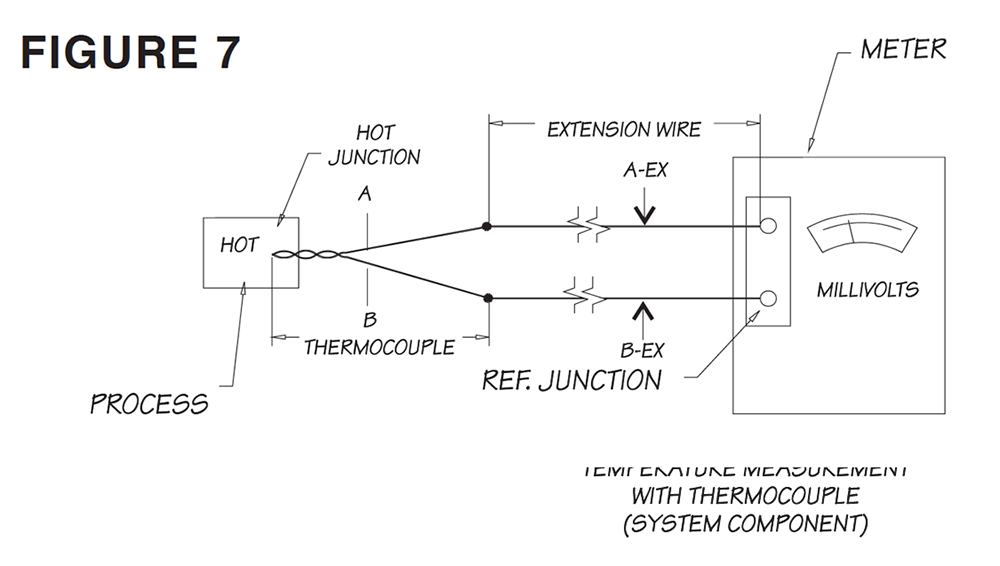# Major Components of Temperature Measurement with Thermocouples1. THERMOCOUPLE

A thermocouple is essentially a simple device, as we have seen, consisting of two dissimilar metals joined together at two junctions. Depending upon the composition of the individual metals in each leg, an “EMF” (millivoltage) is developed which is a function of the difference in temperature between the two junctions of the thermocouple parts and components.

2. PRACTICAL APPROACH

Because a thermocouple sensor generates a voltage that is proportional to temperature differences rather than to absolute temperature, it is necessary to fix one of the junctions at a known temperature for repeatable and accurate measurements. This fixed isothermal connection in thermocouple components is called the reference or the cold junction.

3. EXTENSION WIRE

Most thermocouple installations comprise of a thermocouple, extension wires, and an instrument. Extension wires basically are used to extend thermocouples to an indicator; measuring device or control instruments.

Example: Look at Figure 7.We would like to measure the temperature of the process (We know it is hot, but how hot)? We decided to use type “J” thermocouple wire. Wire A is iron (+), wire B is constantan (-) and we also know that we must use type “J” extension wires. An output of 22.183 millivolts is observed on the measuring instrument. So what do we do with this number? Is our measurement complete? The answer is NO!

Remember in our earlier discussions we indicated that we must “fix” one of the junctions at a known temperature. You know that the process temperature is unknown, therefore, we must know the temperature of the reference point (cold end).

We could very simply use a mercury-in-glass thermometer in the vicinity of the thermocouple reference junction (the cold end) where the instrument is. Let us assume that this mercury thermometer reads 72 degrees Fahrenheit. Now we are in business.

Remember earlier we mentioned that there are tables of temperature versus millivolts for every known standard thermocouple (wires), which are the results of the tests researchers have conducted. Okay, we go to the table for a type “J” thermocouple. Then we go to the temperature column and look for 72 degrees Fahrenheit. In front of 72, in the millivolt column, we will see 1.134 millivolts. OK. That is good. This 1.134 represents 72 degrees Fahrenheit, therefore, our reference point is 72 degrees Fahrenheit. This is the known fixed point. From here on the rest is very easy!

We know the process output was 22.183 millivolts (from the hot junction) and the reference point is 1.134. We simply add these two numbers as follows:

EMF at 72 degrees Fahrenheit                 1.134 mV

Observed EMF                                             22.183 mV

_________

Corrected output                                       23.317 mV

We go back again to the type “J” table in the millivolts column and this time look for 23.317 millivolts. In the temperature column, across from the 23.317 millivolts, we see 800 degrees Fahrenheit. This is the temperature of the process.

We have gone through this simple example to make the following points:

1. We must know how many types of thermocouples are being used today in industry.

2. We must know that there are established tables (temperature vs. millivolts) related to these thermocouples (We will show this table later on).

3. We must know the many cases where extension wires are used and we must be familiar with them.

4. We must know that the instruments that we are using should match the thermocouple type that we intend to use.

5. Most of all, accuracy is NUMBER ONE! We should be aware of the calibration point of the thermocouple. By the previous statement we mean that you just can not use junk thermocouple wire and at the same time expect to have accurate output on your instrumentation output.

If we have the above points in our minds, we can bet that we are on the right track to selecting, designing and applying a reasonable thermocouple system.